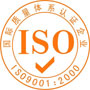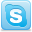### Testing method for Phytic acid 50%

Identification
(1) 3 drops of phenolphthalein solution is added to an aqueous solution (1→10) of this additive, which is neutralized by sodium hydroxide solution. When silver nitrate solution (1→100) is added to this solution, white colloidal precipitates are formed.
(2) 3 ml of sulfuric acid is added to 1 ml of Phytic Acid, which is hydrolyzed by heating for 3 hours in a Kjeldahl flask. To this solution, 3 drops of phenolphthalein solution are added, which is neutralized by sodium hydroxide solution. The resulting solution shows the reaction (2) for Phosphates in Identification.
(3) 7 ml of 30% sulfuric acid is added to 3 ml of Phytic Acid, which is hydrolyzed by heating for 5 hours at 130° in a sealed tube. It is neutralized by sodium hydroxide solution and diluted to 50 ml with water. After adding 0.5 g of activated carbon, it is stirred for 10 minutes and filtered, 30 ml of filtrate is collected. 6 ml of nitric acid is added to 5 ml of this filtrate, which is evaporated to dryness. 0.5 ml of barium chloride solution (1→10) is added to a small portion of the residue. When this is evaporated to dryness in a water bath, the residue becomes pale red color.

Purity
(1) Chlorides : 0.4 g of Phytic Acid is diluted to 10 ml with water, use the Test Solution. This test Solution proceed as directed under chlorides, the content should be equal to or not more than an amount that corresponds to 0.45 ml of 0.01 N hydrochloric acid.
(2) Sulfates : 0.4 g of Phytic Acid is diluted to 10 ml with water, use the Test Solution. This test Solution proceed as directed under sulfates, the content should be equal to or lnot more than an amount that corresponds to 0.6 ml of 0.01 N sulfuric acid.
(3) Arsenic : 0.66 g of Phytic Acid is dissolved in water to make the total volume is to 10 ml. When 5 ml of this test solution proceed as directed under Arsenic, it should be not more than 3ppm.
(5) Free Inorganic Phosphorus : 1.0 g of Phytic Acid is diluted to 500 ml with water. To 3 ml of this solution, 5 ml of ascorbic acid solution (1→100) and 5 ml of molybdate solution, which is 1 g ammonium molybdate in 100 ml of 0.05 N sulfuric acid, are added. The resulting solution is diluted to 50 ml with acetic acid•sodium acetate buffer solution (pH 4.0), which is set aside for 15 minutes ,use the Test Solution. Absorption at 750 nm is measured. Separately, 5 ml of potassium phosphate monobasic standard solution is diluted to 1,000 ml with water. 5 ml each of ascorbic acid solution (1→100) is added to 5.0 ml, 10.0 ml, and 20.0 ml of this solution. Using these solutions, a calibration curve is prepared by following the same procedure as follows. A reference solution is prepared by mixing 5 ml of ascorbic acid solution (1→100) and 5 ml of molybdate solution, which is 1 g ammonium molybdate in 100 ml of 0.05 N sulfuric acid, and diluted to 50 ml with acetic acid•sodium acetate buffer solution (pH 4.0). Using the absorption of Test Solution and the calibration curve, the content of free inorganic phosphorus should be not more than 1.0%

Assay
(1) Total Phosphorus: 1.5 g of Phytic Acid is precisely weighed into a 200 ml Kjeldahl flask, where 10 ml of sulfuric acid and 2.5 ml of nitric acid are added. This is hydrolyzed by heating until the liquid becomes transparent. After cooling, it is diluted to 500 ml with water. 3 ml of this solution is transferred into a 100 ml volumetric flask, neutralized with ammonia water (1→4), and weakly acidified with diluted nitric acid (1→10). 20 ml of metavanadate • molybdate solution are added to the resulting solution, which is diluted to 100 ml with water, mixed by shaking, and set aside for 30 minutes use the Test Solution. Absorption at 420 nm is measured. Separately, 5 ml of potassium phosphate monobasic standard solution is diluted to 1000 ml with water. Using 5.0 ml, 10.0 ml, and 20.0 ml of this solution, a calibration curve is prepared by following the same procedure as Test Solution. Using the absorption of Test Solution and the calibration curve, the content of total phosphorus is obtained.
(2) Bonded Phosphorus : The content of bonded phosphorus is obtained by subtracting free inorganic phosphorus from total phosphorus. The content of phytic acid is obtained from the following equation.
Content of phytic acid (%) = Content of bonded phosphorus(%) × 3.5515
[Solutions]
Metavanadate•molybdate solution : 1.12 g of ammonium metavanadate is dissolved in excess amount of water, where 250 ml of nitric acid is added. 27 g of ammonium molybdate is dissolved in an appropriate amount of water. Two solutions are mixed so that the total volume is 1,000 ml. It is stored in a Light-resistant container.## Products

Phytic acid 50~70%
Solid phytic acid (Purity > 99%)
Sodium Phytate
Calcium Phytate